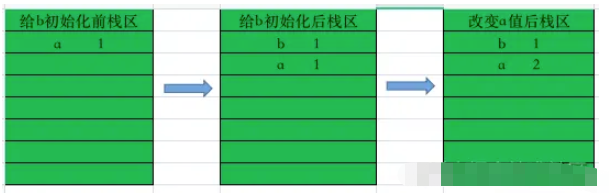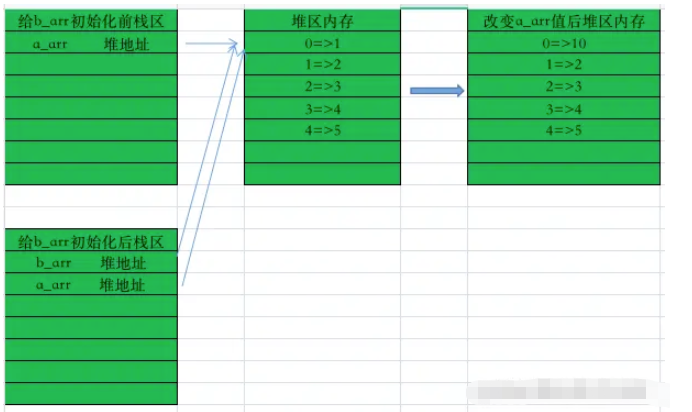# JavaScript引用赋值与传值赋值实例分析

### 前言

• ①具体的数据存在“慢速内存”“堆内存”中；

• ②将变量名和数据地址存在“快速内存”“栈内存”中。

### 基本数据类型

```    var a=1;
var b=a;
a=2;
console.log(b);  // 打印输出结果: 1```### 复合数据类型

```    let a_arr=[1,2,3,4,5];
let b_arr=a_arr;
a_arr=10;
console.log(b_arr); // 打印输出结果: (5) [10, 2, 3, 4, 5]``````    let a_arr=[1,2,3,4,5];
let b_arr=a_arr.concat();
a_arr=10;
console.log(b_arr); // 打印输出结果: (5) [1, 2, 3, 4, 5]```

concat() 方法用于连接两个或多个数组。
concat() 方法不会更改现有数组，而是返回一个新数组，其中包含已连接数组的值。